# 数据结构之红黑树

### 红黑树的定义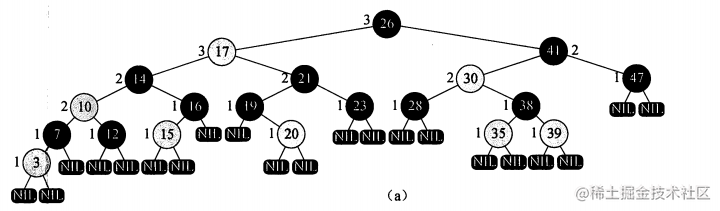### 二叉搜索树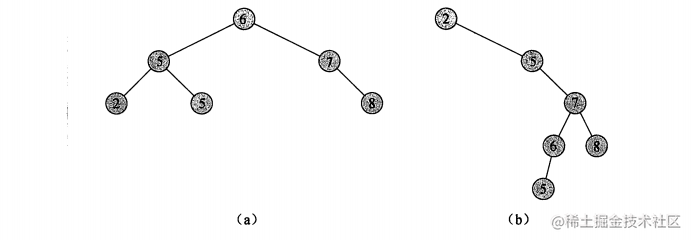### 红黑树的性质

1. 每个节点要么黑色要么红色
2. 根节点必须是黑色
3. 每个叶子节点（叶子结点是NIL结点）是黑色的。（一个结点没有子结点或者父结点，该结点对应相应的指针就指向NIL）
4. 每个红色结点的两个子结点都是黑色的
5. 从任一结点到其每个叶子的所有路径都包含相同数目的黑结点

### 红黑树维持平衡的方式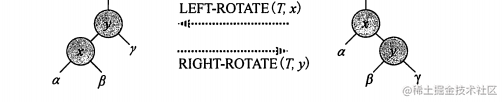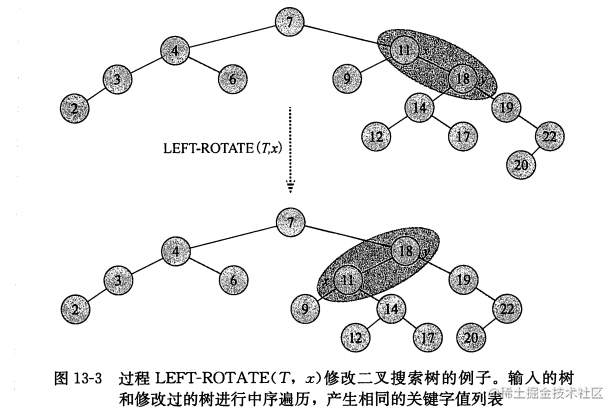### 红黑树的插入

RB-INSERT(T,z)  // T为红黑树、z为要插入的结点
// y设为nil结点
y = T.nil
// x设为根节点
x = T.root
while x != T.nil // x不为空
y = x
if z.key< x.key  // 插入的结点比该树小
x = x.left
else
x = x.right
z.p = y  // 设置插入结点的父结点为 y
if y == T.nil  // 该树为空
T.root = z
else if z.key < y.key
y.left = z
else
y.right = z
// 将插入的结点的左右子结点设为nil
z.left = T.nil
z.right = T.nil
z.color = RED
RB-INSERT-FIXUP(T,z)  // 调整着色

while z.p.color == RED
if z.p == z.p.p.left  // 判断父结点是否是祖父结点的左结点
y = z.p.p.right  // y设为祖父结点的右节点
if y.color = RED  // 判断y的颜色
z.p.color = BLACK  // 将z的父结点的颜色设为黑色  （情况1）
y.color = BLACK  // 将z的叔父结点的颜色设为黑色  （情况1）
z.p.p.color = RED  // 将z的祖父结点的颜色设为红色  （情况1）
z = z.p.p  // 将z结点设为其祖父结点  （情况1）
else if z == z.p.right  // 如果z结点是父结点的右子结点
z = z.p  // 将z结点设为z的父结点  （情况2）
// 对结点进行左旋
LETF-ROTATE(T,z)  （情况2）
z.p.color = BLACK  // 将z的父结点颜色设为黑色  （情况3）
z.p.p.color = RED  // 将z的祖父结点的颜色设为红色  （情况3）
// 对结点进行右旋
RIGHT-ROTATE(T,z.p.p)  （情况3）
else  // 与上面的情况类似
y = z.p.p.left
if y.color = RED
z.p.color = BLACK
y.color = BLACK
z.p.p.color = RED
z = z.p.p
else if z == z.p.left
z = z.p
// 对结点进行左旋
RIGHT-ROTATE(T,z)
z.p.color = BLACK
z.p.p.color = RED
// 对结点进行右旋
LEFT-ROTATE(T,z.p.p)
T.root.color = BLACK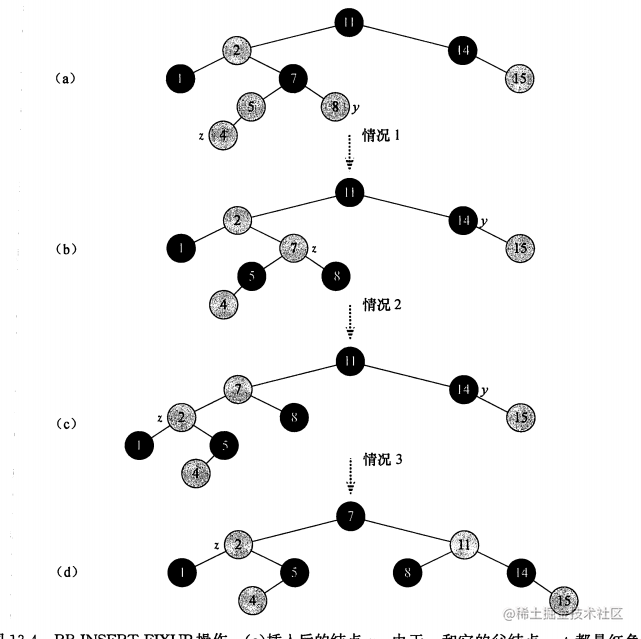### 红黑树的删除

• 无子节点时，删除节点可能为红色或者黑色；
1.1 如果为红色，直接删除即可，不会影响黑色节点的数量；
1.2 如果为黑色，则需要进行删除平衡的操作了；
• 只有一个子节点时，删除节点只能是黑色，其子节点为红色，否则无法满足红黑树的性质了。 此时用删除节点的子节点接到父节点，且将子节点颜色涂黑，保证黑色数量。
• 有两个子节点时，与二叉搜索树一样，使用后继节点作为替换的删除节点，情形转至为1或2处理。